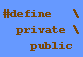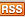### A subset of Python (30 Jun 2013)

Over the years, I collected implementations of an algorithm to solve the subset sum problem in various languages. A few days ago, I experimented a little with Python, and here is the deplorably non-idiomatic and naïve result - apologies offered.

```
<!--
.String { color: #4a708b; }
.Statement { color: #b03060; font-weight: bold; }
.PreProc { color: #1874cd; }
.Constant { color: #ff8c00; }
.Special { color: #8a2be2; }
.Identifier { color: #458b74; }
-->

import random
import array
import sys

numbers = array.array('i')
flags = array.array('c')
solutions = 0

def find_solutions(k, target_sum):
global solutions

if target_sum == 0:
print "  Solution:",
for i in range(0, len(numbers)):
if flags[i] != 0:
print numbers[i],
print
solutions = solutions + 1
else:
if k < len(numbers):
if (numbers[k] * (len(numbers)-k+1)) >= target_sum:
if target_sum >= numbers[k]:
flags[k] = 1
find_solutions(k+1, target_sum - numbers[k])
flags[k] = 0
find_solutions(k+1, target_sum)

def find_subset_sum(target_sum):
global solutions
global flags
print "Subsets which sum up to %s:" % target_sum
flags =  * len(numbers)
find_solutions(0, target_sum)
print "Found", solutions, "different solutions"

def subset_sum_test(size):
global numbers
total = 0

print "Random values:\n  ",
for i in range(0, size):
numbers.append(random.randint(0, 1000))
total = total + numbers[i]
print numbers[i],
print

numbers = sorted(numbers, reverse = True)
target_sum = total/2
find_subset_sum(target_sum)

subset_sum_test(15 if len(sys.argv) < 2 else int(sys.argv))

```

to top

You are here: Blog > WebLeftBar > DefinePrivatePublic201306

r1.1 - 30 Jun 2013 - 12:56 - ClausBrod to topBlog
This site2017: 12 - 11 - 10
2016: 10 - 7 - 3
2015: 11 - 10 - 9 - 4 - 1
2014: 5
2013: 9 - 8 - 7 - 6 - 5
2012: 2 - 10
2011: 1 - 8 - 9 - 10 - 12
2010: 11 - 10 - 9 - 4
2009: 11 - 9 - 8 - 7 -
6 - 5 - 4 - 3
2008: 5 - 4 - 3 - 1
2007: 12 - 8 - 7 - 6 -
5 - 4 - 3 - 1
2006: 4 - 3 - 2 - 1
2005: 12 - 6 - 5 - 4
2004: 12 - 11 - 10
C++
CoCreate Modeling
COM & .NET
Java
Mac
Lisp
OpenSource
Scripting
Windows
Stuff
Changes
Index
Search
Maintenance
Impressum
Datenschutzerklärung
Home

• Webs

Jump:

Copyright © 1999-2023 by the contributing authors. All material on this collaboration platform is the property of the contributing authors.
Ideas, requests, problems regarding TWiki? Send feedback